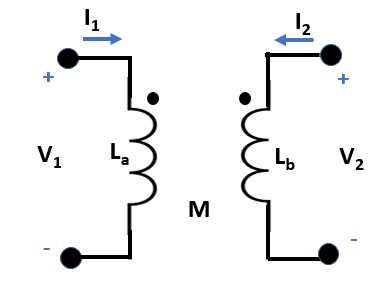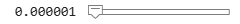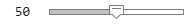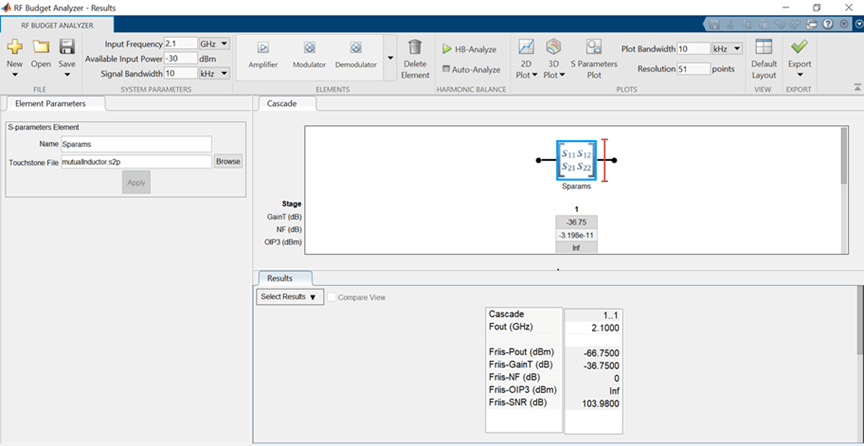# Extract S-Parameters from Mutual Inductor

This example shows how to build a user-defined element from S-parameters and add it to an `rfbudget` object for link budget analysis using the Symbolic Math Toolbox. The user-defined element in this example is a mutual inductor.

Consider a mutual inductor as shown in figure 1 with the inductors ${\mathit{L}}_{\mathit{a}}$and ${\mathit{L}}_{\mathit{b}}$. This examples uses the Symbolic Math Toolbox to extract the analytical S-parameters of the mutual inductor and write them an RF Toolbox™ object. To extract S-parameters from a circuit, see Extract S-Parameters from Circuit.Figure 1: Mutual Inductor

One way to model a mutual inductor in the RF Toolbox is to draw the mutual inductor as an equivalent of a two-port network of inductors in a T configuration. Such a mutual inductor is shown in figure 2 with the mutual inductance $\mathit{M}$ and the coupling coefficient $\mathit{k}$. Mutual inductance is given by the equation $\mathit{M}=\mathit{k}\sqrt{{\mathit{L}}_{\mathit{a}}×{\mathit{L}}_{\mathit{b}}}$ relates M and `k`. Inductors in a T configuration can have negative values when there is a strong coupling between the inductors or if the $\mathit{M}$ is greater than ${\mathit{L}}_{\mathit{a}}$or ${\mathit{L}}_{\mathit{b}}$.Figure 2: T-Circuit Representation of Mutual Inductor

### Represent Circuit in Node-Branch Form

As discussed in the Extract S-Parameters from Circuit example, to extract S-parameters from a circuit you need to drive one port while terminating the other. This is shown in figure 3. Use constitutive and conservative equations to represent the circuit in node-branch form. There are eight unknowns, five branch currents and three node voltages. Therefore there are eight equations in the node-form, five constitutive equations for the branches and three conservative equations obtained from the Kirchoff's Current Law for the nodes. The constitutive equation for a resistor is derived from Ohm's Law,$\mathit{V}=\mathit{IR}$, and the constitutive equation for an inductor is given by $\mathit{V}=\mathit{sLR}$, where `s` is a complex frequency.Figure 3: Mutual Inductor Driven at Port 1 with Current Source

```syms F syms I [5 1] syms V [3 1] syms Z0 La Lb M s nI=5; % number of branch currents nV=3; % number of node voltages % F = [Fconstitutive; Fconservative] F = [ V1 - Z0*I1 V1 - V2 - (La-M)*I3*s V2 - M*I4*s V2 - V3 + (Lb-M)*I5*s V3 - I2*Z0 I1 + I3 I4 - I5 - I3 I2 + I5 ]```
```F =  $\left(\begin{array}{c}{V}_{1}-{I}_{1} {Z}_{0}\\ {V}_{1}-{V}_{2}-{I}_{3} s \left(\mathrm{La}-M\right)\\ {V}_{2}-{I}_{4} M s\\ {V}_{2}-{V}_{3}+{I}_{5} s \left(\mathrm{Lb}-M\right)\\ {V}_{3}-{I}_{2} {Z}_{0}\\ {I}_{1}+{I}_{3}\\ {I}_{4}-{I}_{3}-{I}_{5}\\ {I}_{2}+{I}_{5}\end{array}\right)$```

### Compute Jacobian

Determine the Jacobian with respect to the unknowns, the five branch currents and three node voltages.

`J = jacobian(F,[I; V]);`

### Solve S-parameters for Further Analysis

As shown in the Extract S-Parameter from a Circuit example, create the right-hand side, `rhs` vector to the drive and terminate ports.

```syms rhs [nI+nV 2] syms x v S t % Compute S-parameters of cascade rhs(:,:) = 0; rhs(nI+1,1) = 1/Z0; % rhs for driving input port rhs(nI+nV,2) = 1/Z0 % rhs for driving output port```
```rhs =  $\left(\begin{array}{cc}0& 0\\ 0& 0\\ 0& 0\\ 0& 0\\ 0& 0\\ \frac{1}{{Z}_{0}}& 0\\ 0& 0\\ 0& \frac{1}{{Z}_{0}}\end{array}\right)$```

By backsolving `rhs`, solve for the voltages using Jacobian.

```x = J \ rhs; v = x(nI+[1 nV],:); S = (2*v - eye(2));```

### Create Object for RF Toolbox

In order to create a `sparameters` object, the parameters must be determined at a set of frequencies. To do so, define the variables for your mutual inductor. If you would like to test multiple values for your variables and automatically update your `sparameters` object, use Numeric Sliders in the Control drop-down under the Live Editor tab. Then, use the Symbolic Math Toolbox's `matlabFunction` to automatically generate a function, `mutualInductorS` to compute the analytic S-parameters at a set of frequencies. Finally, use the `sparameters` object to create a S-parameters object.

```matlabFunction(S,'file','mutualInductorS.m','Optimize',false); La =0.000001; Lb =0.000001; Z0 =50; k =0.763; M = k*((La*Lb)^(1/2)); freq = linspace(1e9,2e9,10); s = 2i*pi*freq; s_param = zeros(2,2,10); for index = 1:numel(freq) s_param(:,:,index) = mutualInductorS(Lb,Lb,M,Z0,s(index)); end Sobj = sparameters(s_param,freq);```

### Create Object for RF Budget

Use an `rfwrite` function to create a Touchstone® file from the `sparameters` object.

`rfwrite(Sobj,'mutualInductor.s2p');`

Create a `nport` object.

`n = nport('mutualInductor.s2p');`

Provide the `nport` object as an input to `rfbudget` object.

`b = rfbudget(n,2.1e9,-30,10e3);`

Type this command at the MATLAB Command Window to open the mutual inductor as a S-parameter element in the RF Budget Analyzer app.

```show(b) ```Using this method you can build your own components for RF budget analysis.